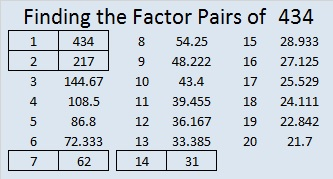# 434 Going Backwards Is Important!

The factors of 434 are listed below the puzzle, but here is a quick problem (really a puzzle) for you to figure out:

434 is the sum of six consecutive prime numbers. One of those primes is 71. What are the other five? If you would like, you may type your answer in the comments.

Solving that problem will require you to work backwards.

Many students may think they understand a mathematical concept, but they don’t really understand it unless they can also do it backwards. For example, they may understand, but maybe not remember that 7 x 8 = 56. Yet, these same students are completely baffled if you tell them this rhyme, “Five, six, seven, eight, 56 is 7 times 8.” They aren’t used to seeing addition, subtraction, or multiplication facts written or spoken in that backwards way. Coleen Young made an excellent slide presentation titled good-mathematicians-can-go-backwards. We ALL can go forward in mathematics so much more confidently once we learn to go backwards.

Coleen recommends many resources including the Find The Factors puzzles. Each of these puzzles can be solved and then completely filled out as a multiplication table if you will go backwards first and find the factors of the clues in the grid. Think what two numbers multiplied together give you 49? Give it a try!

Print the puzzles or type the factors on this excel file: 12 Factors 2015-03-23

• 434 is a composite number.
• Prime factorization: 434 = 2 x 7 x 31
• The exponents in the prime factorization are 1, 1, and 1. Adding one to each and multiplying we get (1 + 1)(1 + 1)(1 + 1) = 2 x 2 x 2 = 8. Therefore 434 has exactly 8 factors.
• Factors of 434: 1, 2, 7, 14, 31, 62, 217, 434
• Factor pairs: 434 = 1 x 434, 2 x 217, 7 x 62, or 14 x 31
• 434 has no square factors that allow its square root to be simplified. √434 ≈ 20.8327